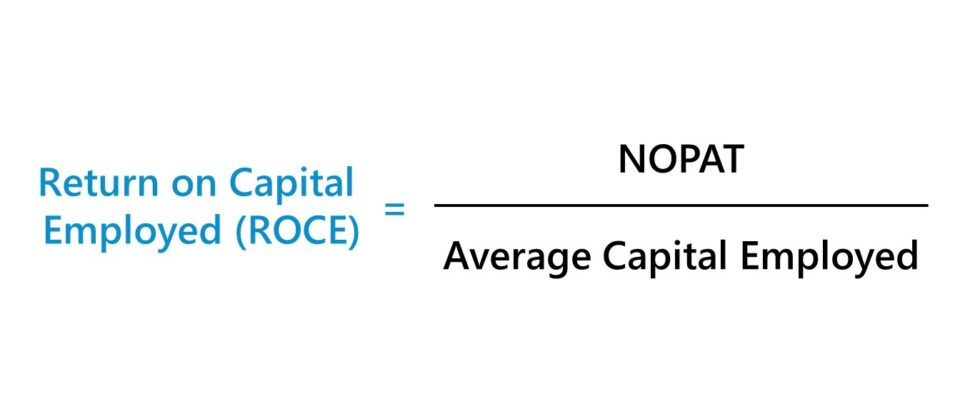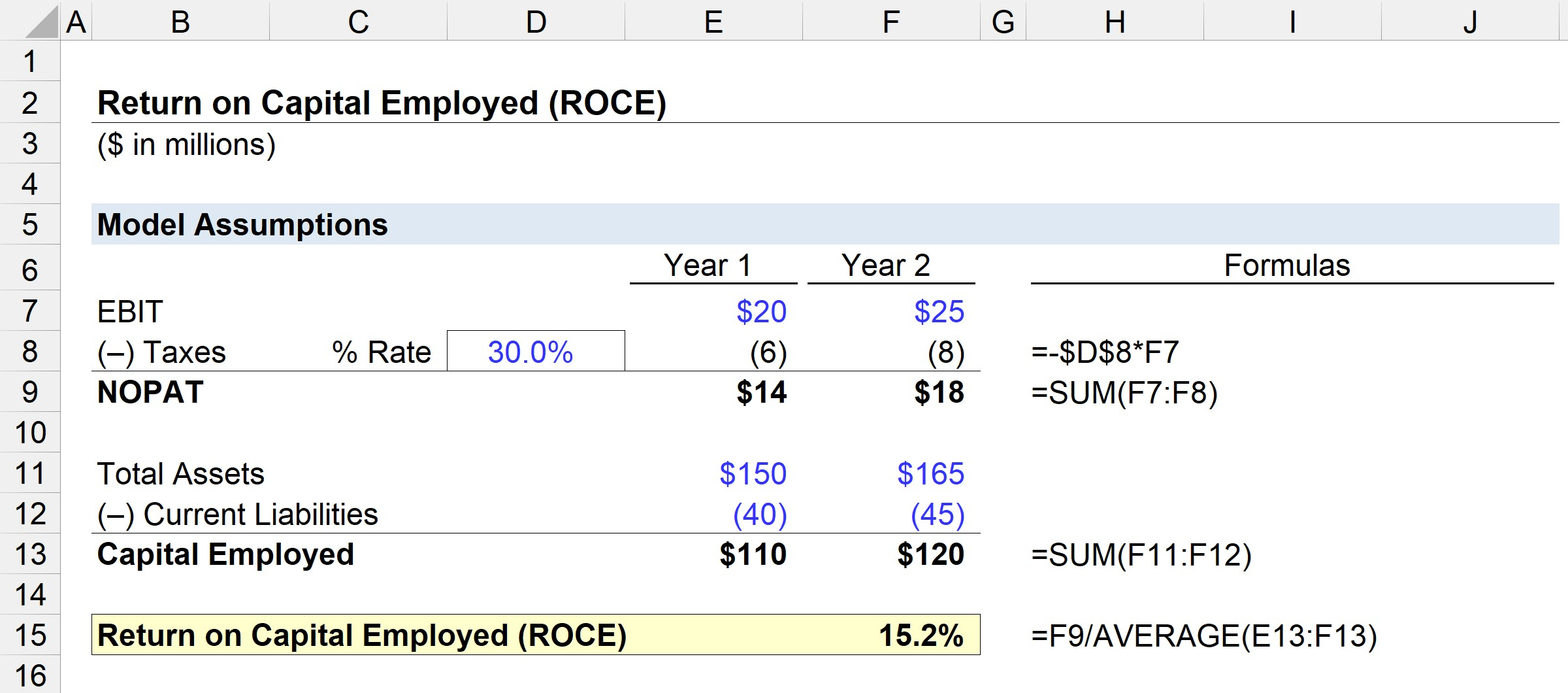Welcome to Wall Street Prep! Use code at checkout for 15% off.# Return on Capital Employed (ROCE)

Guide to Understanding Return on Capital Employed (ROCE)## How to Calculate ROCE?

ROCE, shorthand for “Return on Capital Employed,” is a profitability ratio comparing a profit metric to the amount of capital employed.

The return on capital employed (ROCE) metric answers the question of, “How much in profits does the company generate for each dollar in capital employed?”

Given a ROCE of 10%, the interpretation is that the company generates \$1.00 of profits for each \$10.00 in capital employed.

ROCE can be a useful proxy for operational efficiency, particularly for capital-intensive industries.

• Telecom and Communications
• Oil & Gas
• Industrials and Transportation
• Manufacturing

The calculation of return on capital employed is a two-step process, starting with the calculation of net operating profit after taxes (NOPAT).

NOPAT, also known as “EBIAT” (i.e. earnings before interest after taxes), is the numerator, which is subsequently divided by capital employed.

NOPAT = EBIT × (1 Tax Rate %)

The denominator, capital employed, is equal to the sum of shareholders’ equity and long-term debts, i.e. total assets less current liabilities.

Capital Employed = Total Assets Current Liabilities

More specifically, all the assets sitting on a company’s balance sheet – i.e. the resources with positive economic value – were originally funded somehow, either using equity or debt (i.e. the accounting equation).

If we deduct current liabilities, we are removing the non-financing liabilities from total assets (e.g. accounts payable, accrued expenses, deferred revenue).

That said, the capital employed encompasses shareholders’ equity, as well as non-current liabilities, namely long-term debt.

Capital Employed = Shareholders’ Equity + Non-Current Liabilities

## ROCE Formula

The formula for calculating the return on capital employed (ROCE) metric is as follows.

Return on Capital Employed (ROCE) = NOPAT ÷ Capital Employed

In contrast, certain calculations of ROCE use operating income (EBIT) in the numerator, as opposed to NOPAT.

Since profits paid out in the form of taxes are not available to financiers, one can argue that EBIT should be tax-affected, resulting in NOPAT.

Regardless, ROCE is unlikely to deviate much whether EBIT or NOPAT is used, although be sure to maintain consistency in any comparisons or calculations.

## What is a Good ROCE?

Generally speaking, the higher a company’s return on capital employed (ROCE), the better off the company likely is with regard to generating long-term profits.

• Higher ROCE → Implies the capital employment strategies of a company are more efficient
• Lower ROCE → Potential signal that the company is spending funds unproductively (i.e. there is substantial “waste” in capital allocation)

The average ROCE will vary by industry, so comparisons must be done among peer groups comprised of similar companies to determine whether a given company’s ROCE is “good” or “bad”.

The current ROCE of a company can also be viewed in relation to that of its historical periods to assess the consistency at which capital is efficiently deployed.

Stability in maintaining a high ROCE year after year can build the case that a company possesses an economic moat and can achieve excess returns on capital over the long run.

## How to Interpret ROCE?

Often, return on capital employed (ROCE) is compared to the weighted average cost of capital (WACC) – i.e. the required rate of return and hurdle rate – to determine which projects/investments to accept or reject.

• If ROCE > WACC = “Accept”
• If ROCE < WACC = “Reject”

But as usual, reliance on a single metric is not recommended, so ROCE should be supplemented with other metrics such as the return on invested capital (ROIC), which we’ll expand upon in the next section.

## What is the Difference Between ROCE vs. ROIC?

The return on capital employed (ROCE) and return on invested capital (ROIC) are two closely related measures of profitability.

ROIC represents the percentage return earned by a company, accounting for the amount of capital invested by equity and debt providers. Both ROCE and ROIC determine the efficiency at which the capital on hand is allocated by a company.

ROCE = NOPAT ÷ Average Capital Employed
ROIC = NOPAT ÷ Average Invested Capital

Consistent ROCE and ROIC metrics are likely to be perceived positively, as the company appears to be spending its capital efficiently.

The distinction between ROCE and ROCE is in the denominator – i.e. capital employed vs invested capital.

• Capital Employed = Total Assets – Current Liabilities
• Invested Capital = PP&E + Net Working Capital (NWC)

For ROCE, capital employed captures the total amount of debt financing and equity available to fund operations and purchase assets.

On the other hand, ROIC uses invested capital – which is equal to fixed assets (i.e. property, plant & equipment, or “PP&E”) plus net working capital (NWC).

## ROCE Calculator

We’ll now move to a modeling exercise, which you can access by filling out the form below.#### Excel TemplateSubmitting...

## 1. Operating Assumptions

In our illustrative scenario, we will be using the following assumptions.

Year 1 Financial Data:

• EBIT = \$20 million
• Total Assets = \$150 million
• Current Liabilities = \$40 million

Year 2 Financial Data:

• EBIT = \$25 million
• Total Assets = \$165 million
• Current Liabilities = \$45 million

## 2. NOPAT and Capital Employed Calculation Example

Assuming that the tax rate for both periods is 30.0%, NOPAT can be calculated by multiplying EBIT by one minus the tax rate assumption.

• NOPAT, Year 1 = \$20 million × (1 – 30%) = \$14 million
• NOPAT, Year 2 = \$25 million × (1 – 30%) = \$18 million

The next step is to calculate the capital employed, which is equal to total assets minus current liabilities.

• Capital Employed, Year 1 = \$150 million – \$40 million = \$110 million
• Capital Employed, Year 2 = \$165 million – \$45 million = \$120 million

From Year 1 to Year 2, NOPAT grew from \$14 million to \$18 million, while the capital employed rose from \$110 million to \$120 million under the same time frame.

## 3. ROCE Calculation Example

If we input those figures into the return on capital employed (ROCE) formula, the ROCE of our example company comes out to 15.2%.

• Return on Capital Employed (ROCE) = \$18 million ÷ (\$110 million + \$120 million ÷ 2) = 15.2%

The 15.2% ROCE means that we can estimate that for each \$10 of capital employed, \$1.52 is returned as profits, which can be compared to the rate of industry peers and historical periods to determine if management is efficient at capital utilization.Step-by-Step Online Course

### Everything You Need To Master Financial Modeling

Enroll in The Premium Package: Learn Financial Statement Modeling, DCF, M&A, LBO and Comps. The same training program used at top investment banks.

Inline Feedbacks
Joseph
May 20, 2023 6:39 am

thanks so much for the help really needed it